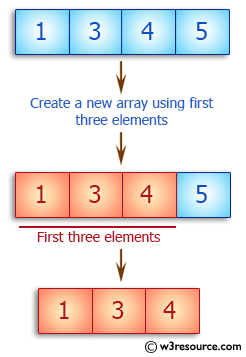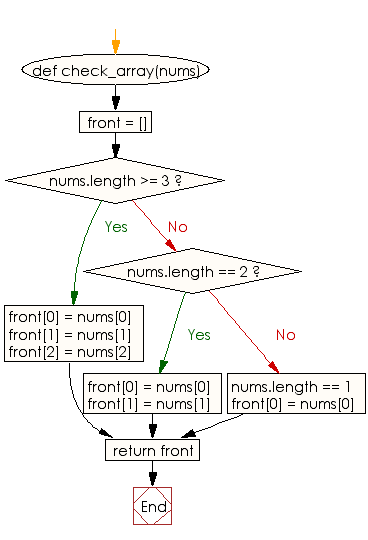﻿ Ruby Array exercises: Create a new array using first three elements of a given array of integers - w3resource# Ruby Array Exercises: Create a new array using first three elements of a given array of integers

## Ruby Array: Exercise-27 with Solution

Write a Ruby program to create a new array using first three elements of a given array of integers. If the length of the given array is less than three return the original array.Ruby Code:

``````def check_array(nums)
front = []
if nums.length >= 3
front = nums
front = nums
front = nums
elsif nums.length == 2
front = nums
front = nums
else nums.length == 1
front = nums
end
return front
end

print check_array([1, 3, 4, 5]),"\n"
print check_array([1, 2, 3]),"\n"
print check_array([1,2]),"\n"
print check_array(),"\n"
``````

Output:

```[1, 3, 4]
[1, 2, 3]
[1, 2]

```

Flowchart:Ruby Code Editor: